# Problems Based on Ages : Download Reasoning Study Notes For DSSSB Exam## Study Notes on Problem Based on Ages

Equation Problems of Age are part of the Reasoning Ability Section. At least 2-3 questions are asked from equation problems of ages topic in most of the exams. These equations could become either linear or non-linear. To solve these types of questions candidates require the knowledge of linear equation. This method needs some basic concepts. Today we are going to provide you some important tips for the Problems Based on Ages questions.

Are You Preparing For DSSSB Exam? Grab This Opportunity Now.. ENROLL NOW

Have a look at the following questions:

Ex. 1: The age of the father 3 years ago was 7 times the age of his son. At present, the father’s age is five times that of his son. What are the present ages of the father and the son?

Ex. 2: At present, the age of the father is five times the age of his son. Three years hence, the father’s age would be four times that of his son. Find the present ages of the father and the son.

Ex. 3: Three years earlier, the father was 7 times as old as his son. Three years hence, the father’s age would be four times of his son. What are the present ages of the father and the son?

Ex. 4: The sum of the ages of a mother and her daughter is 50 yrs. Also 5 years ago, the mother’s age was 7 times the age of the daughter. What are the present ages of the mother and the daughter?

Ex. 5: The sum of the ages of a son and father is 56 yrs. After 4 years, the age of the father will be three times that of the son. What is the age of the son?

Ex. 6: The ratio of the ages of the father and the son at present is 6: 1. After 5 years, the ratio will become 7:2. What is the present age of the son?

BY THE CONVENTIONAL METHOD:
Solution: 1. Let the present age of son = x years
Then, the present age of father = 5x yr
3 years ago,
7(x -3) = 5x – 3
Or, 7x k- 21 = 5x – 3
Or, 2x = 18
x = 9 years
Therefore, son’s age = 9 years
Father’s age = 45 years

Solution: 2. Let the present age of son = x years
Then, the present age of father = 5x years
3 years hence,
4(x + 3) = 5x + 3
Or, 4x + 12 = 5x + 3
x = 9 yrs.
Therefore, son’s age = 9 yrs and father’s age = 45 years

Solution : 3. Let the present age of son = x yrs and the present ag of father = y yrs
3 yrs earlier, 7(x – 3) = y – 3 Or, 7x – y – 18 . . . . . . . . . . . .(i)
3 yrs hence, 4(x + 3) = y + 3
Or, 4x + 12 = y + 3 Or, 4x – y = 9 . . . . . . . . . . . .(ii)
Solving (1) & (2) we get, x = 9 yrs & y = 45 yrs

Check Reasoning Section Syllabus for DSSSB Exam

Solution : 4. Let the age of the daughter be x yrs.
Then, the age of the mother is (50x – x)yrs
5 yrs ago, 7(x – 5) = 50 – x – 5
Or, 8x = 50 – 5 + 35 = 80
x = 10
Therefore, daughter’s age = 10 yrs and mother’s age = 40 yrs

Solution : 5. Let the age of the son be x yrs.
Then, the age of the father is (56 – x) yrs.
After 4 yrs, 3(x + 4) = 56 – x + 4
Or, 4x = 56 + 4 – 12 = 48
x = 12 yrs
Thus, son’s age = 12 yrs.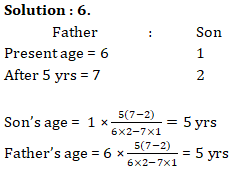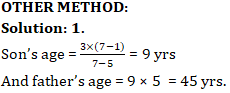Undoubtedly you get confused with the above method, but it is very easy to understand are remembered. See the following form of question.

#### Practice More Reasoning Quizzes with DSSSB Study Plan

Question : 1 yrs earlier the father’s age was x times that of his son. At present the father’s age is y times that of his son. What are the present ages of the son and the father ?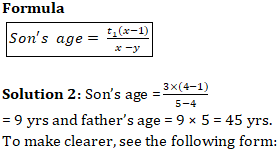Questions : The present age of the father is y times the age of his son. t2 yrs hence, the father’s age become z times the age of his son. What are the present ages of the father and his son ?
Formula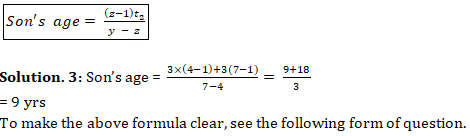Questions : t1 yrs earlier, the age of the father was x times the age of his son. t2 yrs hence, the age of the father becomes z times the age of his son. What are the present ages of the son and the father ?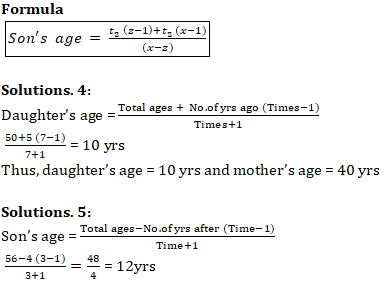Note : Do you get the similarities between the above two direct methods? They differ only in sign in the numerator. When the question deals with ‘ago’, a +ve sign exists and when it deals with ‘after’ a -ve sign exists in the numerator.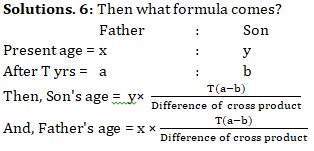Note:
1. While evaluating the difference of cross-product, always take +ve sign.
2. Both the above direct formulas look similar. The only difference you can find is in the denominators. But it has been simplified as “difference of cross-products to make it easier to remember. So, with the help of one formula only you can solve both the question.
3. We suggest you to go through both the methods and choose the better of the two.
4. We hope that the post would have cleared all your doubts related to the topic.